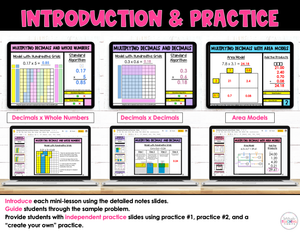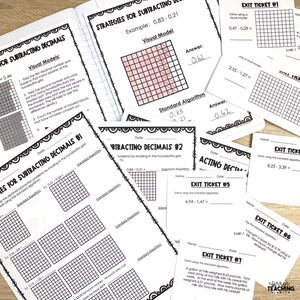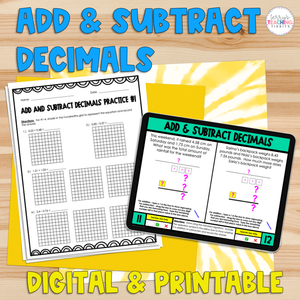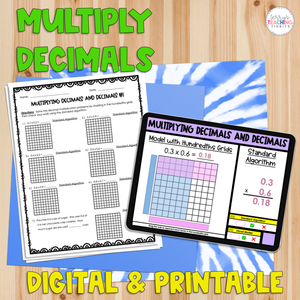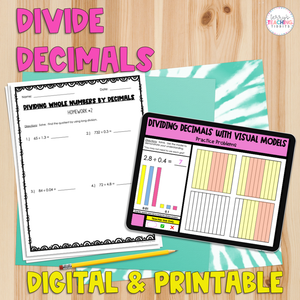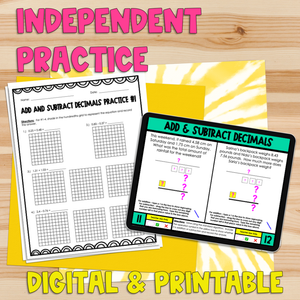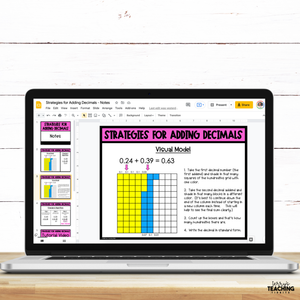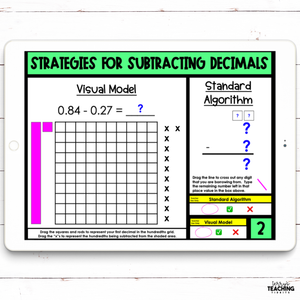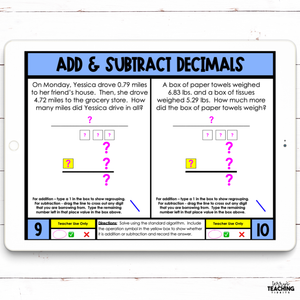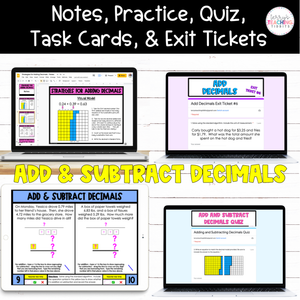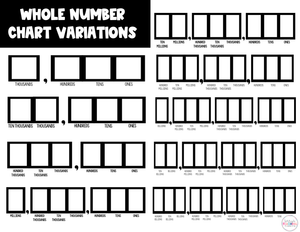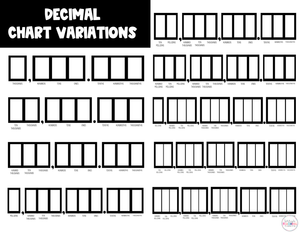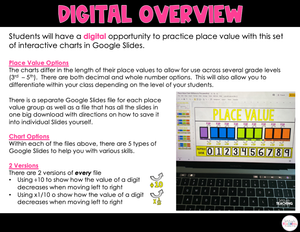# Dividing Decimals Digital Resource Pack {with Visual Models}

Regular price
\$12.00
Regular price
Sale price
\$12.00
Unit price
per
Availability
Sold out
Shipping calculated at checkout.

This resource provides digital instructional materials for teaching students how to divide decimals. Perfect for distance learning!

The resource is divided into 6 sections of mini-lessons based on the type of decimal division problem you’re teaching:
• dividing decimals by whole numbers
• dividing whole numbers by decimals
• dividing decimals by decimals (with the same # of decimal places in the dividend and divisor)
• dividing decimals by decimals (with a different # of decimal places in the dividend and divisor)
• dividing decimals by decimals in which you have to annex a zero in the dividend
• dividing decimals by decimals using a visual model

## What You Get

In Each Section:
• Digital Guided Notes (Google Slides)
• a Video tutorial
• 4 classwork activities (Google Slides)
• Exit tickets to assess your students quickly throughout the unit (Google Forms)